# Question 13 1 pts Multiplication in the time domain is: 1st derivative in frequency domain Addition...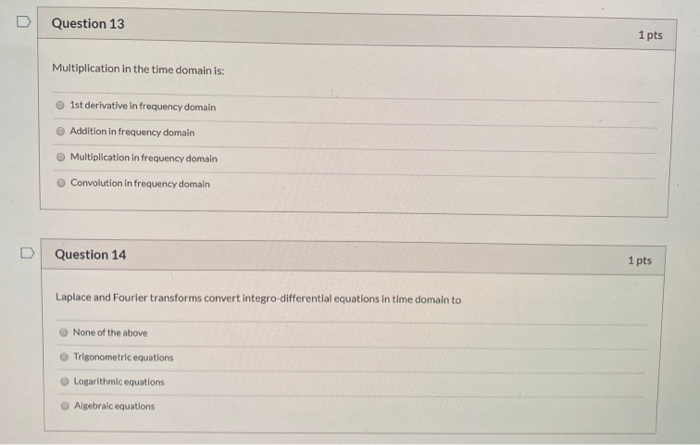Question 13 1 pts Multiplication in the time domain is: 1st derivative in frequency domain Addition in frequency domain Multiplication in frequency domain Convolution in frequency domain Question 14 1 pts Laplace and Fourier transforms convert integro-differential equations in time domain to None of the above Trigonometric equations Logarithmic equations Algebraic equations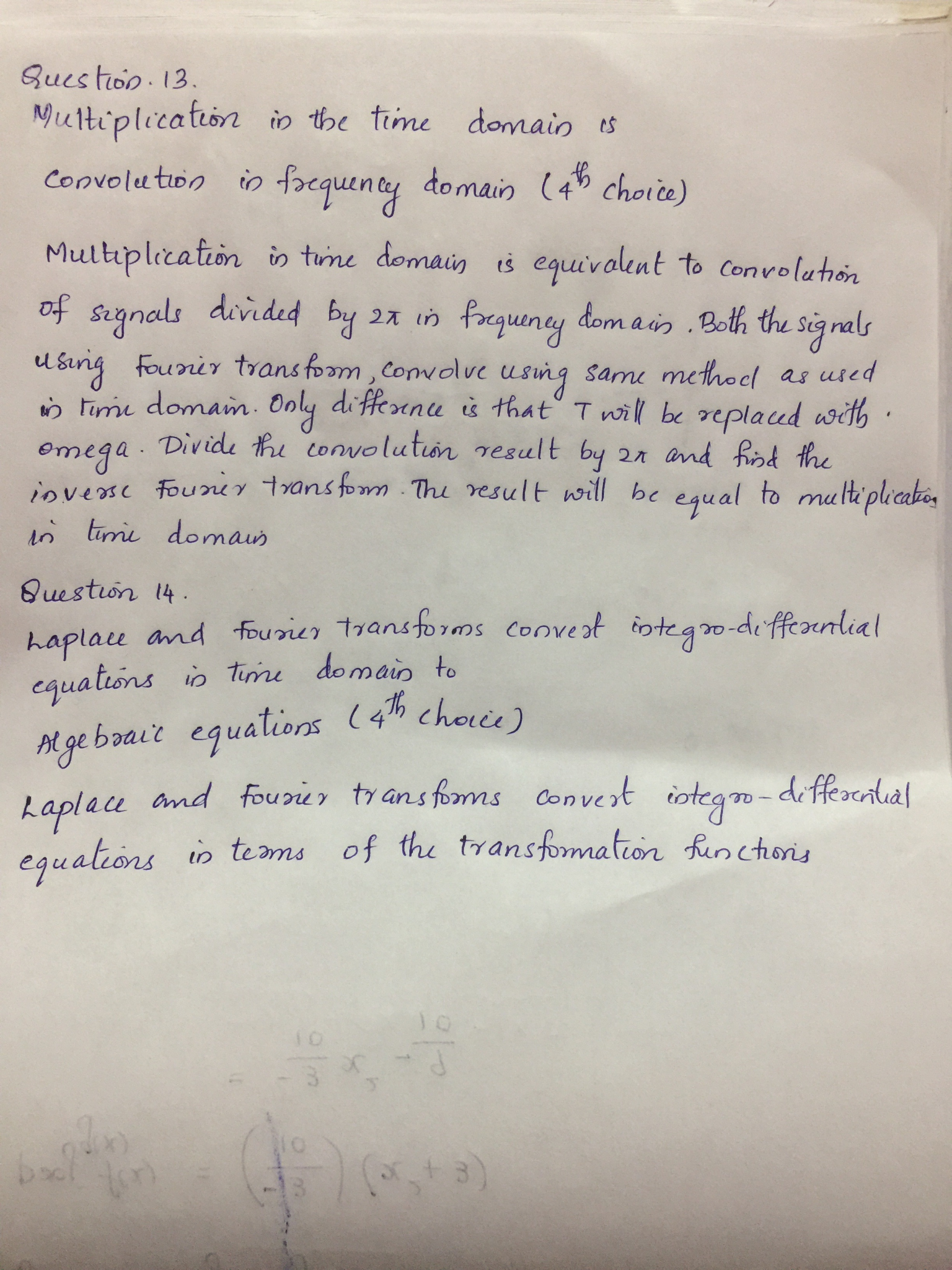##### Add Answer of: Question 13 1 pts Multiplication in the time domain is: 1st derivative in frequency domain Addition...
Similar Homework Help Questions
• ### Question 35 1 pts Shift in time domain results in: Convolution in frequency domain Multiplication by...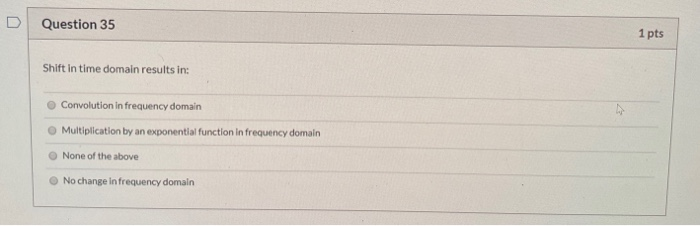Question 35 1 pts Shift in time domain results in: Convolution in frequency domain Multiplication by an exponential function in frequency domain None of the above No change in frequency domain

• ### Question 12 Frequency shift in S domain results in: None of the above 1st derivative in...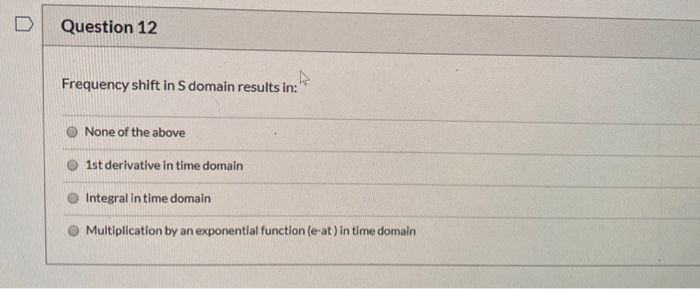Question 12 Frequency shift in S domain results in: None of the above 1st derivative in time domain Integral in time domain Multiplication by an exponential function (e-at) in time domain

• ### Question 9 1 pts The convolution of 2 time domain signals is a function of Impulse...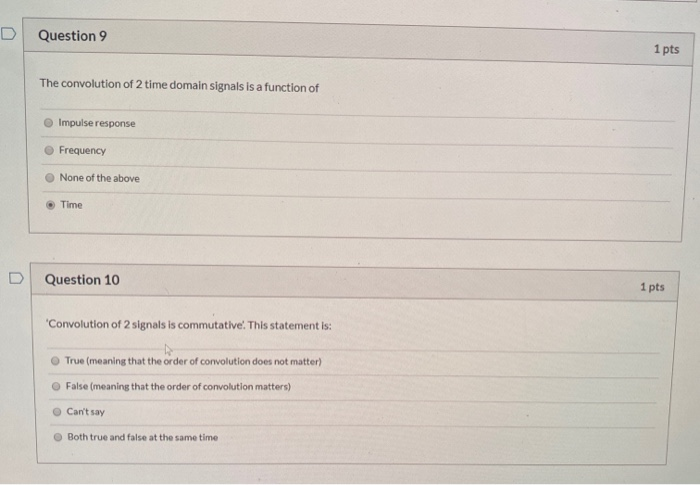Question 9 1 pts The convolution of 2 time domain signals is a function of Impulse response Frequency None of the above Time Question 10 1 pts Convolution of 2 signals is commutative. This statement is: True (meaning that the order of convolution does not matter) False (meaning that the order of convolution matters) Can't say Both true and false at the same time

• ### Question 33 1 pts Fourier transform of the impulse response of a system is: Same as...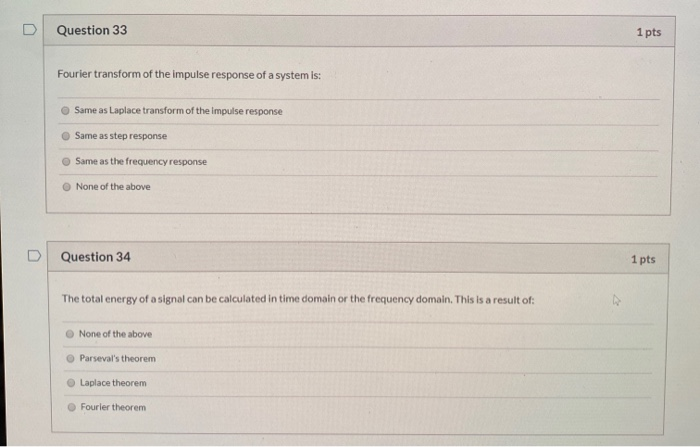Question 33 1 pts Fourier transform of the impulse response of a system is: Same as Laplace transform of the Impulse response Same as step response Same as the frequency response None of the above Question 34 1 pts The total energy of a signal can be calculated in time domain or the frequency domain. This is a result of: None of the above Parseval's theorem Laplace theorem Fourier theorem

• ### The total energy of a signal can be calculated in time domain or the frequency domain....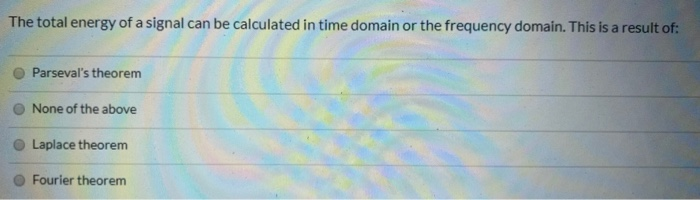The total energy of a signal can be calculated in time domain or the frequency domain. This is a result of: Parseval's theorem None of the above Laplace theorem Fourier theorem

• ### Question 11 1 pts An LTI system is BIBO stable if and only if the impulse...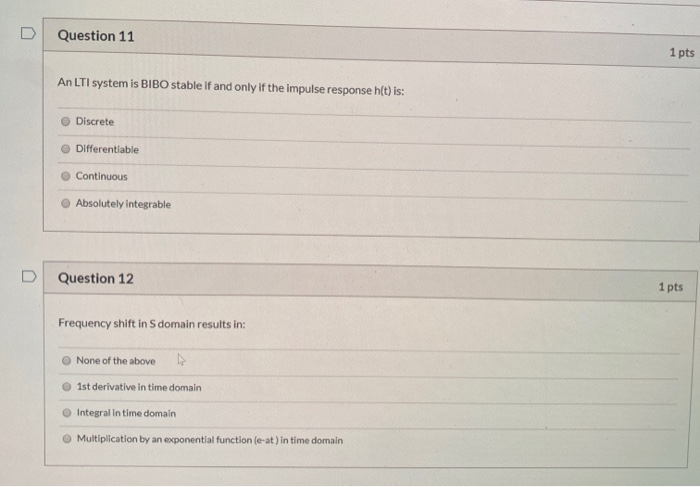Question 11 1 pts An LTI system is BIBO stable if and only if the impulse response h(t) is: Discrete Differentiable Continuous Absolutely integrable Question 12 1 pts Frequency shift in S domain results in: None of the above Ist derivative in time domain Integral in time domain Multiplication by an exponential function (e-at) in time domain

• ### Question 29 1 pts Fourier series analysis is applied to: Non periodic functions Tangential functions Periodic...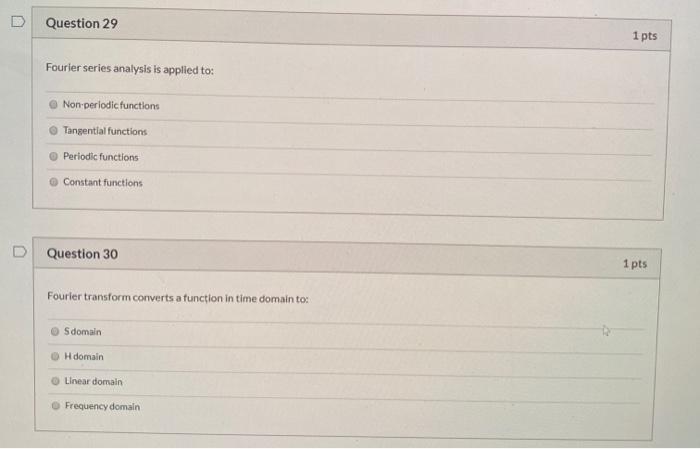Question 29 1 pts Fourier series analysis is applied to: Non periodic functions Tangential functions Periodic functions Constant functions Question 30 1 pts Fourier transform converts a function in time domain to: S domain OH domain Linear domain Frequency domain

• ### Question 27 1 pts Fourier transform of a rectangular pulse is a: Sine function Tan function...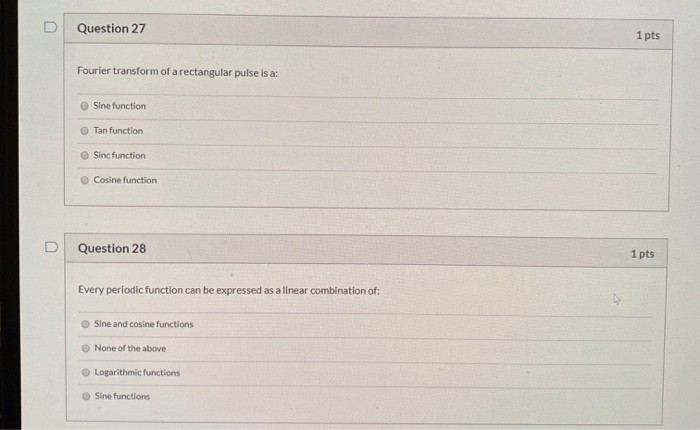Question 27 1 pts Fourier transform of a rectangular pulse is a: Sine function Tan function Sinc function Cosine function D Question 28 1 pts Every periodic function can be expressed as a linear combination of: Sine and cosine functions None of the above Logarithmic functions Sine functions

• ### QUESTION 1 Consider the time domain signal shown below. Determine the magnitude of the Fourier Tr...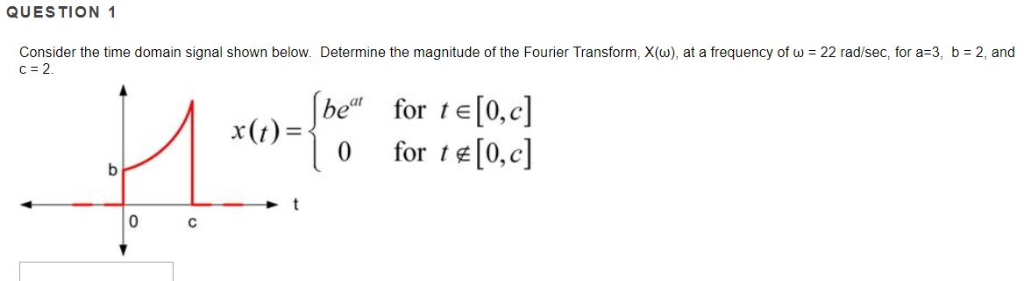QUESTION 1 Consider the time domain signal shown below. Determine the magnitude of the Fourier Transform, X(w), at a frequency of ω : 22 rad/sec, for a-3, b = 2, and c=2 be" for te[0,c] x(t)-| 0 forte(0,c] QUESTION 1 Consider the time domain signal shown below. Determine the magnitude of the Fourier Transform, X(w), at a frequency of ω : 22 rad/sec, for a-3, b = 2, and c=2 be" for te[0,c] x(t)-| 0 forte(0,c]

• ### Question 24 1 pts Using the shooting method for the following second-order differential equation governing the...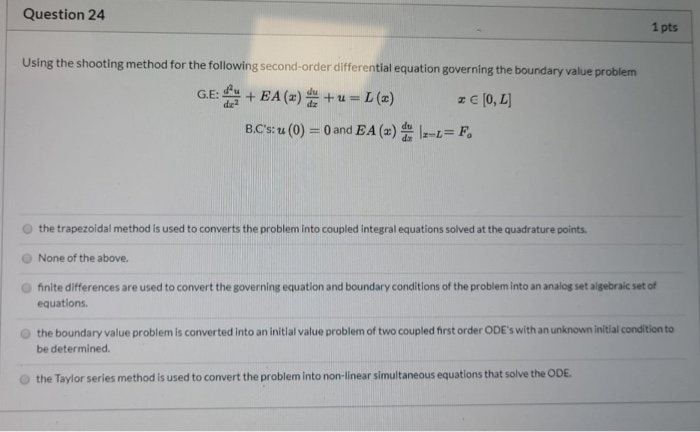Question 24 1 pts Using the shooting method for the following second-order differential equation governing the boundary value problem G.E: + EA (x) +u = L (x) € (0,L] B.C's: u () = 0 and EA (2) de Iz-L=F, the trapezoidal method is used to converts the problem into coupled integral equations solved at the quadrature points. None of the above. finite differences are used to convert the governing equation and boundary conditions of the problem into an analog set...

Free Homework App